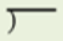# Access Point #: MAFS.4.NBT.2.AP.6a

Find whole-number quotients and remainders with up to three-digit dividends and one-digit divisors, using two different strategies.
Clarifications:

#### Essential Understandings

Concrete:

• Given an expression, identify the dividend and the divisor (dividend = the total amount, divisor = number of groups or the number in each group).
• Use base ten blocks to model the dividend.
• Use base ten blocks to divide into groups determined by the divisor in order to find the quotient.
Representation:
• Symbols ÷, =,• Understand the following concepts and vocabulary of division, part, whole, divisor, dividend, quotient.
• Given a division expression, find the array using a jig on a pictorial representation to solve.
General Information
Number: MAFS.4.NBT.2.AP.6a
Category: Access Points
Cluster: Use place value understanding and properties of operations to perform multi-digit arithmetic. (Major Cluster)

Clusters should not be sorted from Major to Supporting and then taught in that order. To do so would strip the coherence of the mathematical ideas and miss the opportunity to enhance the major work of the grade with the supporting clusters.

## Related Standards

This access point is an alternate version of the following benchmark(s).

## Related Courses

This access point is part of these courses.
5012015: Foundational Skills in Mathematics 3-5

## Related Resources

Vetted resources educators can use to teach the concepts and skills in this access point.